# RS Aggarwal Solutions for Class 7 Maths Exercise 4F Chapter 4 Rational Numbers

RS Aggarwal Solutions for Class 7 Maths Exercise 4F Chapter 4 Rational Numbers in simple PDF are given here. The topics covered in this exercise are the reciprocal or multiplicative inverse of a rational number and division of rational numbers. Students will go through this RS Aggarwal Solutions for Class 7 Maths Chapter 4 Rational Numbers thoroughly with more practice. Students can hence score good marks in Maths by practising RS Aggarwal Class 7 Solutions.

## Download the PDF of RS Aggarwal Solutions For Class 7 Maths Chapter 4 Rational Numbers – Exercise 4F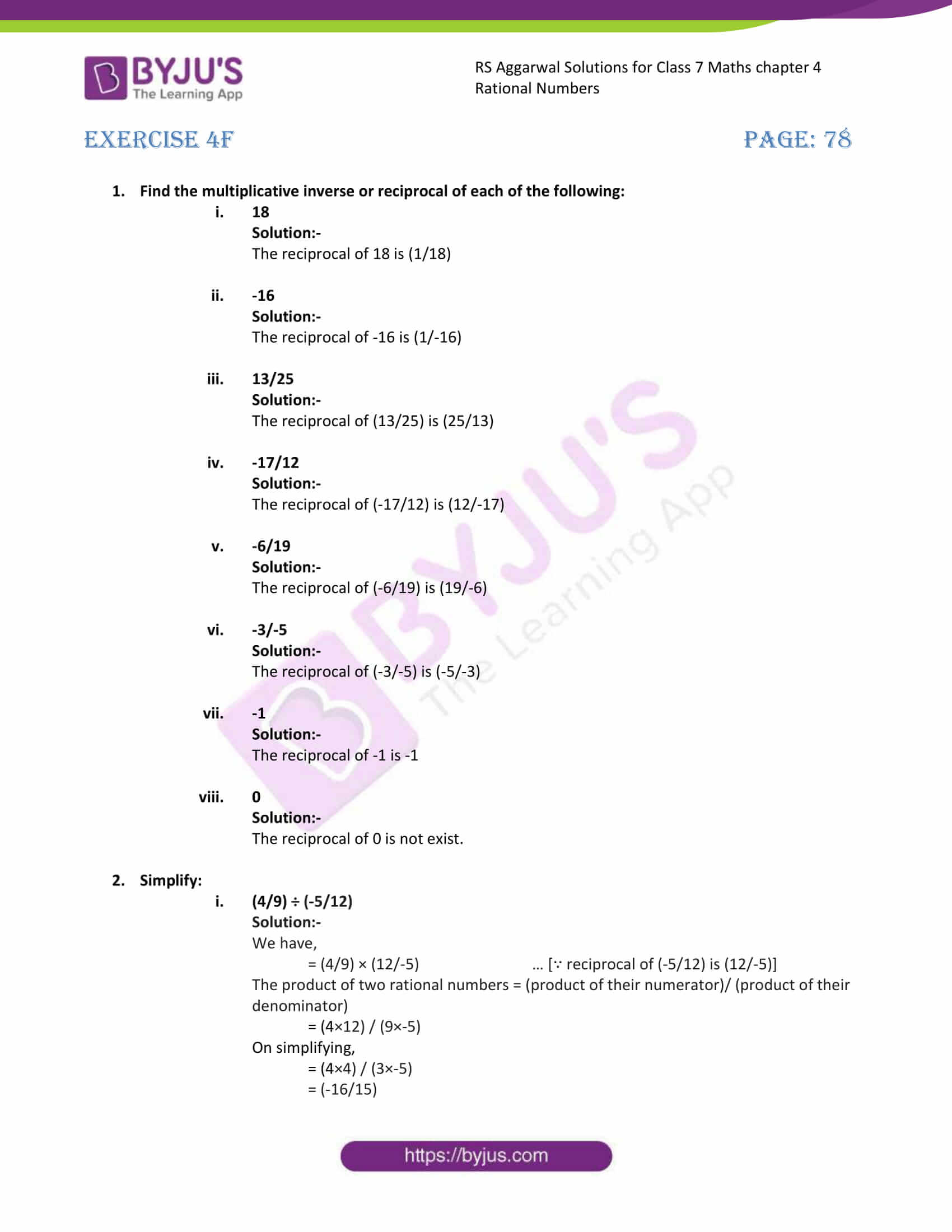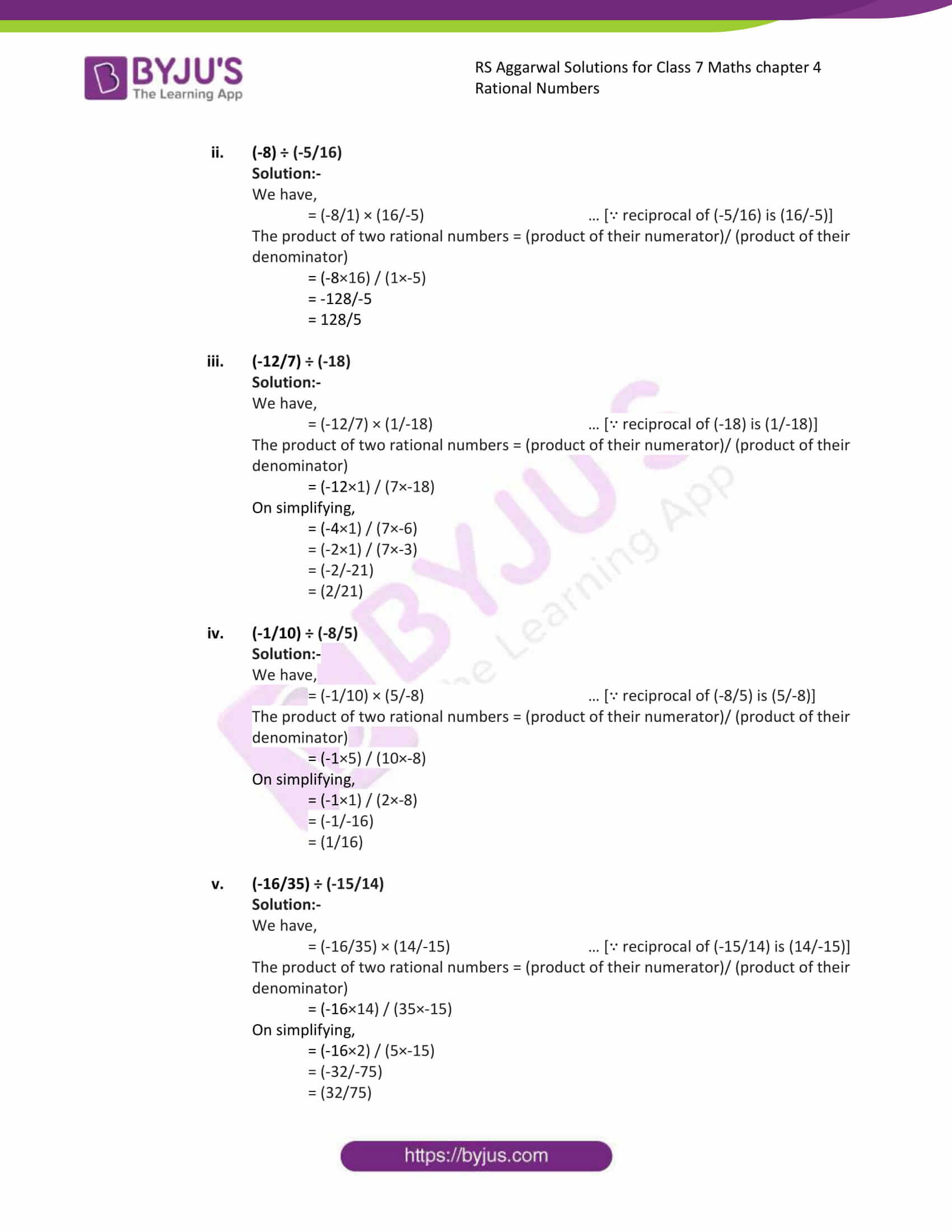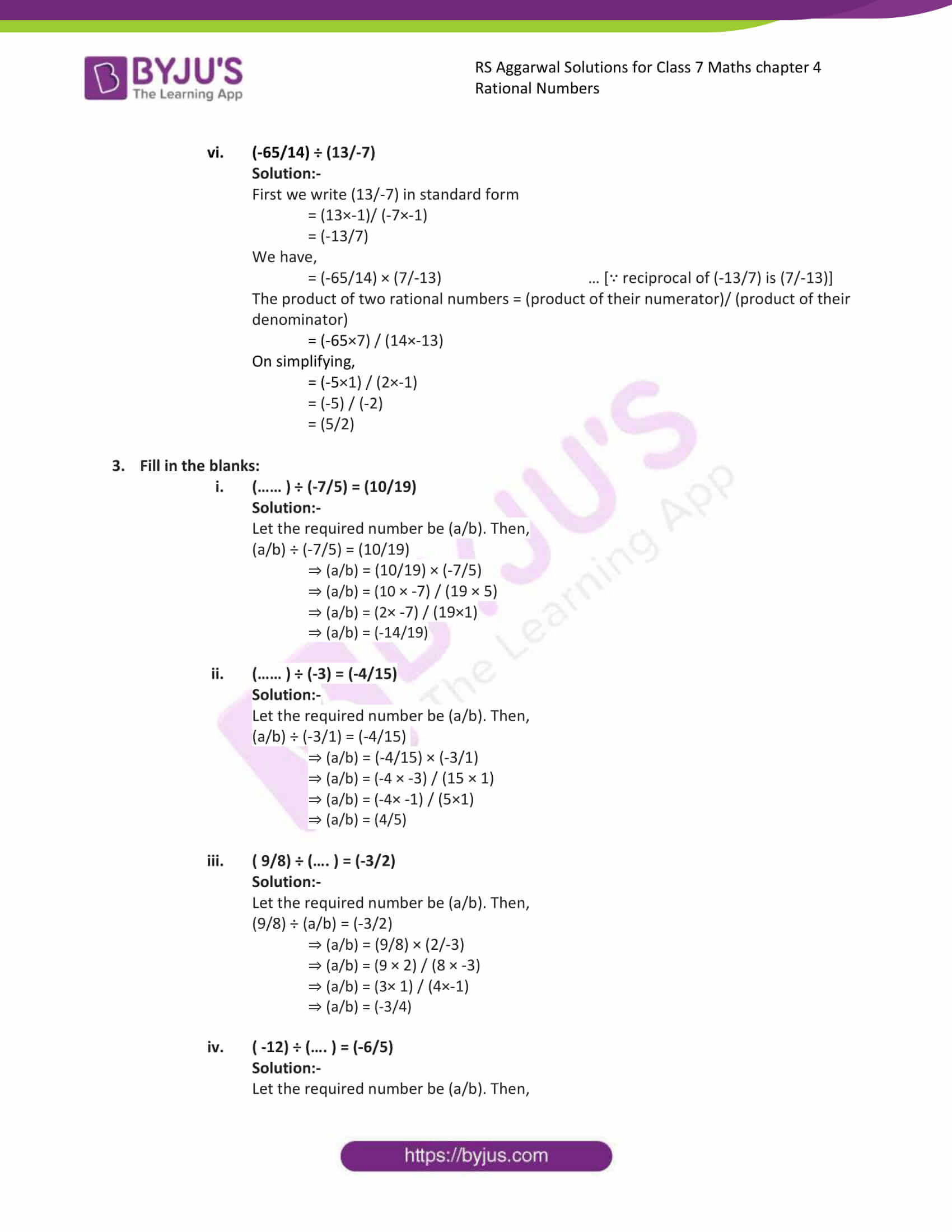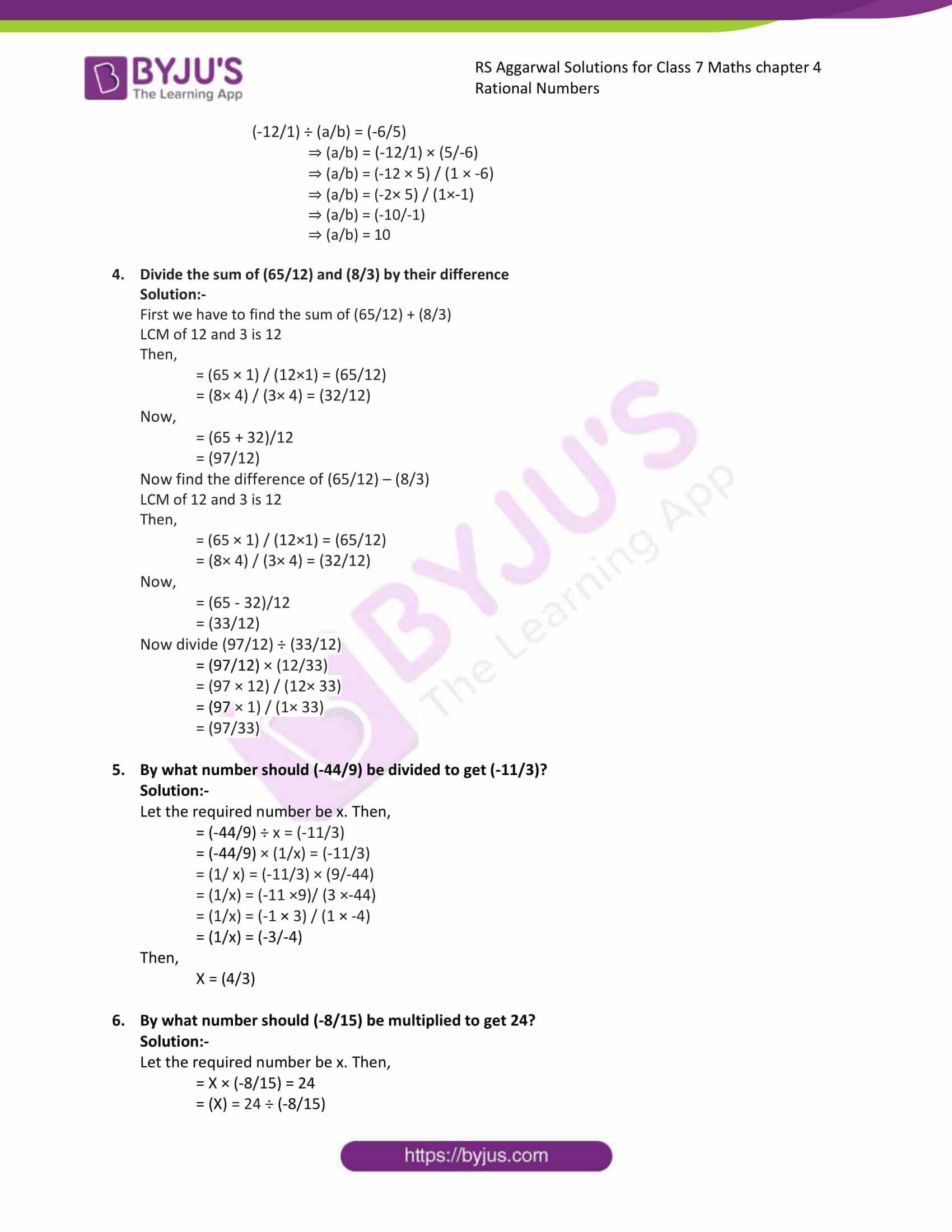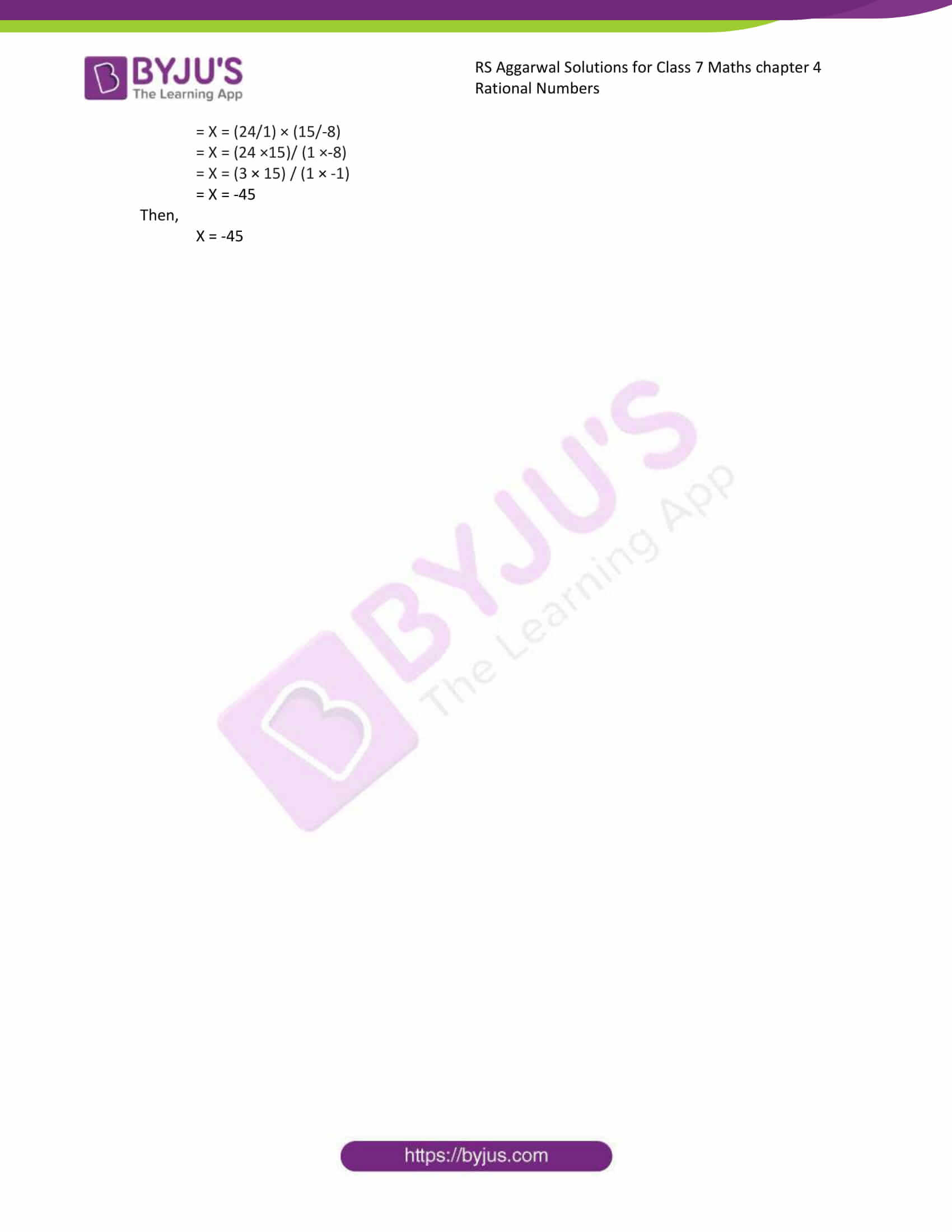### Access answers to Maths RS Aggarwal Solutions for Class 7 Chapter 4 – Rational Numbers Exercise 4F

1. Find the multiplicative inverse or reciprocal of each of the following:
(i) 18

Solution:-

The reciprocal of 18 is (1/18)

(ii) -16

Solution:-

The reciprocal of -16 is (1/-16)

(iii) 13/25

Solution:-

The reciprocal of (13/25) is (25/13)

(iv) -17/12

Solution:-

The reciprocal of (-17/12) is (12/-17)

(v) -6/19

Solution:-

The reciprocal of (-6/19) is (19/-6)

(vi) -3/-5

Solution:-

The reciprocal of (-3/-5) is (-5/-3)

(vii) -1

Solution:-

The reciprocal of -1 is -1

(viii) 0

Solution:-

The reciprocal of 0 is not exist.

2. Simplify:
(i) (4/9) ÷ (-5/12)

Solution:-

We have,

= (4/9) × (12/-5) … [∵ reciprocal of (-5/12) is (12/-5)]

The product of two rational numbers = (product of their numerator)/ (product of their denominator)

= (4×12) / (9×-5)

On simplifying,

= (4×4) / (3×-5)

= (-16/15)

(ii) (-8) ÷ (-5/16)

Solution:-

We have,

= (-8/1) × (16/-5) … [∵ reciprocal of (-5/16) is (16/-5)]

The product of two rational numbers = (product of their numerator)/ (product of their denominator)

= (-8×16) / (1×-5)

= -128/-5

= 128/5

(iii) (-12/7) ÷ (-18)

Solution:-

We have,

= (-12/7) × (1/-18) … [∵ reciprocal of (-18) is (1/-18)]

The product of two rational numbers = (product of their numerator)/ (product of their denominator)

= (-12×1) / (7×-18)

On simplifying,

= (-4×1) / (7×-6)

= (-2×1) / (7×-3)

= (-2/-21)

= (2/21)

(iv) (-1/10) ÷ (-8/5)

Solution:-

We have,

= (-1/10) × (5/-8) … [∵ reciprocal of (-8/5) is (5/-8)]

The product of two rational numbers = (product of their numerator)/ (product of their denominator)

= (-1×5) / (10×-8)

On simplifying,

= (-1×1) / (2×-8)

= (-1/-16)

= (1/16)

(v) (-16/35) ÷ (-15/14)

Solution:-

We have,

= (-16/35) × (14/-15) … [∵ reciprocal of (-15/14) is (14/-15)]

The product of two rational numbers = (product of their numerator)/ (product of their denominator)

= (-16×14) / (35×-15)

On simplifying,

= (-16×2) / (5×-15)

= (-32/-75)

= (32/75)

(vi) (-65/14) ÷ (13/-7)

Solution:-

First we write (13/-7) in standard form

= (13×-1)/ (-7×-1)

= (-13/7)

We have,

= (-65/14) × (7/-13) … [∵ reciprocal of (-13/7) is (7/-13)]

The product of two rational numbers = (product of their numerator)/ (product of their denominator)

= (-65×7) / (14×-13)

On simplifying,

= (-5×1) / (2×-1)

= (-5) / (-2)

= (5/2)

3. Fill in the blanks:
(i) (…… ) ÷ (-7/5) = (10/19)

Solution:-

Let the required number be (a/b). Then,

(a/b) ÷ (-7/5) = (10/19)

⇒ (a/b) = (10/19) × (-7/5)

⇒ (a/b) = (10 × -7) / (19 × 5)

⇒ (a/b) = (2× -7) / (19×1)

⇒ (a/b) = (-14/19)

(ii) (…… ) ÷ (-3) = (-4/15)

Solution:-

Let the required number be (a/b). Then,

(a/b) ÷ (-3/1) = (-4/15)

⇒ (a/b) = (-4/15) × (-3/1)

⇒ (a/b) = (-4 × -3) / (15 × 1)

⇒ (a/b) = (-4× -1) / (5×1)

⇒ (a/b) = (4/5)

(iii) ( 9/8) ÷ (…. ) = (-3/2)

Solution:-

Let the required number be (a/b). Then,

(9/8) ÷ (a/b) = (-3/2)

⇒ (a/b) = (9/8) × (2/-3)

⇒ (a/b) = (9 × 2) / (8 × -3)

⇒ (a/b) = (3× 1) / (4×-1)

⇒ (a/b) = (-3/4)

(iv) ( -12) ÷ (…. ) = (-6/5)

Solution:-

Let the required number be (a/b). Then,

(-12/1) ÷ (a/b) = (-6/5)

⇒ (a/b) = (-12/1) × (5/-6)

⇒ (a/b) = (-12 × 5) / (1 × -6)

⇒ (a/b) = (-2× 5) / (1×-1)

⇒ (a/b) = (-10/-1)

⇒ (a/b) = 10

4. Divide the sum of (65/12) and (8/3) by their difference

Solution:-

First we have to find the sum of (65/12) + (8/3)

LCM of 12 and 3 is 12

Then,

= (65 × 1) / (12×1) = (65/12)

= (8× 4) / (3× 4) = (32/12)

Now,

= (65 + 32)/12

= (97/12)

Now find the difference of (65/12) – (8/3)

LCM of 12 and 3 is 12

Then,

= (65 × 1) / (12×1) = (65/12)

= (8× 4) / (3× 4) = (32/12)

Now,

= (65 – 32)/12

= (33/12)

Now divide (97/12) ÷ (33/12)

= (97/12) × (12/33)

= (97 × 12) / (12× 33)

= (97 × 1) / (1× 33)

= (97/33)

5. By what number should (-44/9) be divided to get (-11/3)?

Solution:-

Let the required number be x. Then,

= (-44/9) ÷ x = (-11/3)

= (-44/9) × (1/x) = (-11/3)

= (1/ x) = (-11/3) × (9/-44)

= (1/x) = (-11 ×9)/ (3 ×-44)

= (1/x) = (-1 × 3) / (1 × -4)

= (1/x) = (-3/-4)

Then,

X = (4/3)

6. By what number should (-8/15) be multiplied to get 24?

Solution:-

Let the required number be x. Then,

= X × (-8/15) = 24

= (X) = 24 ÷ (-8/15)

= X = (24/1) × (15/-8)

= X = (24 ×15)/ (1 ×-8)

= X = (3 × 15) / (1 × -1)

= X = -45

Then,

X = -45

### Access other exercises of RS Aggarwal Solutions For Class 7 Chapter 4 – Rational Numbers

Exercise 4G Solutions